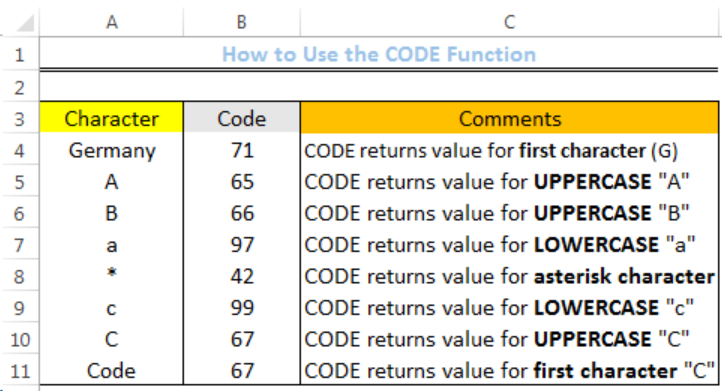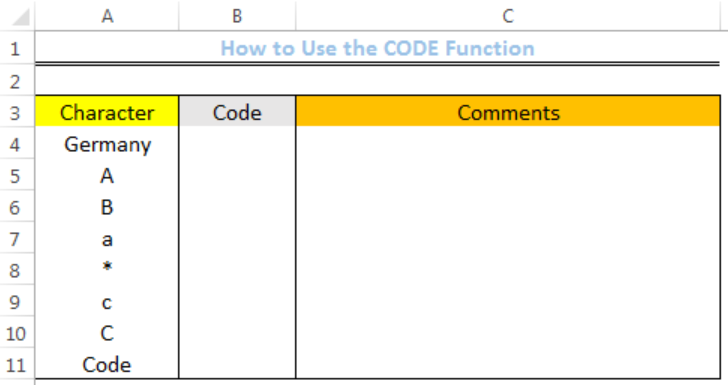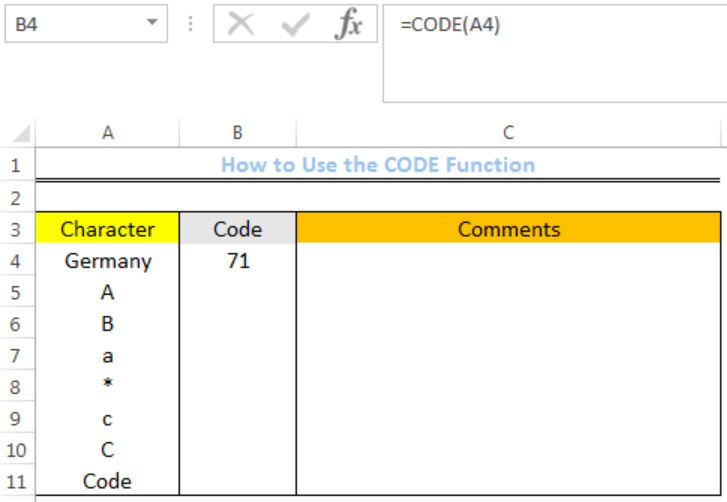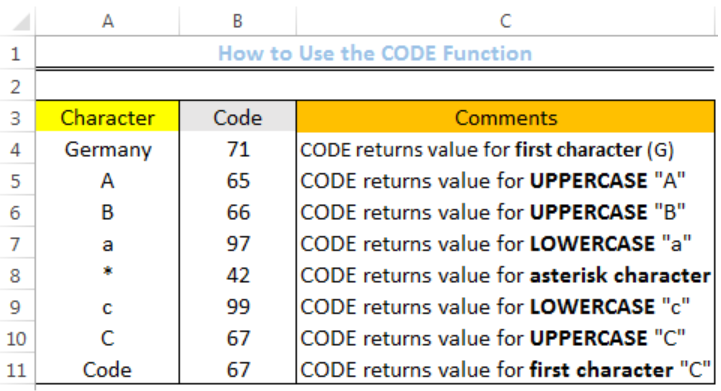Get instant live expert help with Excel or Google Sheets“My Excelchat expert helped me in less than 20 minutes, saving me what would have been 5 hours of work!”

#### Post your problem and you’ll get expert help in seconds.

Your message must be at least 40 characters
Our professional experts are available now. Your privacy is guaranteed.

# How to Use the Excel CODE Function

We can use the Excel CODE FUNCTION to return a numeric code for a given character. The CODE function generates a numeric code for the first character in a text string. The code is based on the character set used by our computer. The steps below will walk through the process.Figure 1: How to Use the Excel CODE Function

## Syntax

`=CODE(TEXT)`

## Formula

`=CODE(A4)`

## Setting up the Data

• We will set up the data by inputting the Characters in Column A
• Column B is where the formula will return the Numeric code for the characters in Column AFigure 2: Setting up the Data

## Excel CODE Function

• We will click on Cell B4
• We will insert the formula below into the cell
`=CODE(A4)`
• We will press the enter keyFigure 3: Returned Numeric Code for the First Character (G) in Cell A4

• We will click on Cell B4 again
• We will double-click on the fill handle (the small plus sign at the bottom right of Cell B4) and drag down to copy the formula into the other cellsFigure 4: Returned Numeric Codes

## Instant Connection to an Expert through our Excelchat Service

Most of the time, the problem you will need to solve will be more complex than a simple application of a formula or function. If you want to save hours of research and frustration, try our live Excelchat service! Our Excel Experts are available 24/7 to answer any Excel question you may have. We guarantee a connection within 30 seconds and a customized solution within 20 minutes.

### Did this post not answer your question? Get a solution from connecting with the expert.Another blog reader asked this question today on Excelchat:
Solution examplesI need shorthand to reference the column in the row im working on. For instance instead of writing =SUMIF(Table2[ITEM CODE],B8,Table2[QTY]) I want to write something like =SUMIF(Table2[ITEM CODE],Column(B),Table2[QTY])
Solved by C. C. in 20 minsGuest
Jim Graham

I am trying to set up a calulation to limit the amount of calculation that needs to be done by an individual. I am looking for a formula that would allow me to put in a number (where the ball was on the field) and then auto calculate the yards gained by the previous spot. The problem that i am having is calculating from the 10 to the 50 yard line is easy, however when going over to the 50 and say putting the ball on the other 30, what would that formula look like. example ball on own 30… Read more »

Comment awaiting moderation

## Subscribe to Excelchat.coAnother blog reader asked this question today on Excelchat: